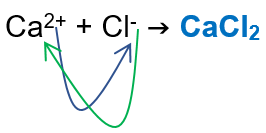# Problem: Calculate the molarity of an aqueous solution that is 18.2% by mass calcium chloride. You might need to know that the density is 1.18 g/mL.

###### FREE Expert Solution

We’re being asked to calculate the molarity (M) of an 18.2% by mass calcium chloride (CaCl2) solution. Recall that molarity is the ratio of the moles of solute and the volume of solution (in liters). In other words:

We first need to determine the number of moles of calcium chloride:

Calcium → Group 2A → +2 charge → Ca2+
Chloride → Group 7A → -1 charge → Cl-We’re given the mass percent of calcium chloride (CaCl2) in water, 18.2%. Recall that mass percent is given by:###### Problem Details

Calculate the molarity of an aqueous solution that is 18.2% by mass calcium chloride. You might need to know that the density is 1.18 g/mL.